Intermediate Algebra

# 9.2Solve Quadratic Equations by Completing the Square

Intermediate Algebra9.2 Solve Quadratic Equations by Completing the Square

### Learning Objectives

By the end of this section, you will be able to:
• Complete the square of a binomial expression
• Solve quadratic equations of the form $x2+bx+c=0x2+bx+c=0$ by completing the square
• Solve quadratic equations of the form $ax2+bx+c=0ax2+bx+c=0$ by completing the square

### Be Prepared 9.2

Before you get started, take this readiness quiz.

1. Expand: $(x+9)2.(x+9)2.$
If you missed this problem, review Example 5.32.
2. Factor $y2−14y+49.y2−14y+49.$
If you missed this problem, review Example 6.9.
3. Factor $5n2+40n+80.5n2+40n+80.$
If you missed this problem, review Example 6.14.

So far we have solved quadratic equations by factoring and using the Square Root Property. In this section, we will solve quadratic equations by a process called completing the square, which is important for our work on conics later.

### Complete the Square of a Binomial Expression

In the last section, we were able to use the Square Root Property to solve the equation (y − 7)2 = 12 because the left side was a perfect square.

$(y−7)2=12y−7=±12y−7=±23y=7±23(y−7)2=12y−7=±12y−7=±23y=7±23$

We also solved an equation in which the left side was a perfect square trinomial, but we had to rewrite it the form $(x−k)2(x−k)2$ in order to use the Square Root Property.

$x2−10x+25=18(x−5)2=18x2−10x+25=18(x−5)2=18$

What happens if the variable is not part of a perfect square? Can we use algebra to make a perfect square?

Let’s look at two examples to help us recognize the patterns.

$(x+9)2(y−7)2 (x+9)(x+9)(y−7)(y−7) x2+9x+9x+81y2−7y−7y+49 x2+18x+81y2−14y+49(x+9)2(y−7)2 (x+9)(x+9)(y−7)(y−7) x2+9x+9x+81y2−7y−7y+49 x2+18x+81y2−14y+49$

We restate the patterns here for reference.

### Binomial Squares Pattern

If a and b are real numbers,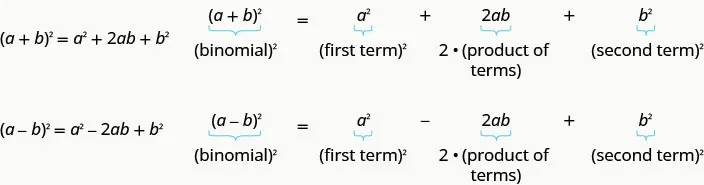We can use this pattern to “make” a perfect square.

We will start with the expression x2 + 6x. Since there is a plus sign between the two terms, we will use the (a + b)2 pattern, a2 + 2ab + b2 = (a + b)2.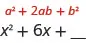We ultimately need to find the last term of this trinomial that will make it a perfect square trinomial. To do that we will need to find b. But first we start with determining a. Notice that the first term of x2 + 6x is a square, x2. This tells us that a = x.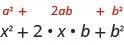What number, b, when multiplied with 2x gives 6x? It would have to be 3, which is $12(6).12(6).$ So b = 3.Now to complete the perfect square trinomial, we will find the last term by squaring b, which is 32 = 9.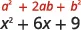We can now factor.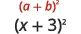So we found that adding 9 to x2 + 6x ‘completes the square’, and we write it as (x + 3)2.

### How To

#### Complete a square of $x2+bx.x2+bx.$

1. Step 1. Identify b, the coefficient of x.
2. Step 2. Find $(12b)2,(12b)2,$ the number to complete the square.
3. Step 3. Add the $(12b)2(12b)2$ to x2 + bx.
4. Step 4. Factor the perfect square trinomial, writing it as a binomial squared.

### Example 9.11

Complete the square to make a perfect square trinomial. Then write the result as a binomial squared.

$x2−26xx2−26x$ $y2−9yy2−9y$ $n2+12nn2+12n$

### Try It 9.21

Complete the square to make a perfect square trinomial. Then write the result as a binomial squared.

$a2−20aa2−20a$ $m2−5mm2−5m$ $p2+14pp2+14p$

### Try It 9.22

Complete the square to make a perfect square trinomial. Then write the result as a binomial squared.

$b2−4bb2−4b$ $n2+13nn2+13n$ $q2−23qq2−23q$

### Solve Quadratic Equations of the Form x2 + bx + c = 0 by Completing the Square

In solving equations, we must always do the same thing to both sides of the equation. This is true, of course, when we solve a quadratic equation by completing the square too. When we add a term to one side of the equation to make a perfect square trinomial, we must also add the same term to the other side of the equation.

For example, if we start with the equation x2 + 6x = 40, and we want to complete the square on the left, we will add 9 to both sides of the equation.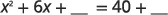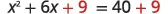Add 9 to both sides to complete the square.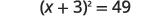Now the equation is in the form to solve using the Square Root Property! Completing the square is a way to transform an equation into the form we need to be able to use the Square Root Property.

### Example 9.12

#### How to Solve a Quadratic Equation of the Form $x2+bx+c=0x2+bx+c=0$ by Completing the Square

Solve by completing the square: $x2+8x=48.x2+8x=48.$

### Try It 9.23

Solve by completing the square: $x2+4x=5.x2+4x=5.$

### Try It 9.24

Solve by completing the square: $y2−10y=−9.y2−10y=−9.$

The steps to solve a quadratic equation by completing the square are listed here.

### How To

#### Solve a quadratic equation of the form $x2+bx+c=0x2+bx+c=0$ by completing the square.

1. Step 1. Isolate the variable terms on one side and the constant terms on the other.
2. Step 2. Find $(12·b)2,(12·b)2,$ the number needed to complete the square. Add it to both sides of the equation.
3. Step 3. Factor the perfect square trinomial, writing it as a binomial squared on the left and simplify by adding the terms on the right
4. Step 4. Use the Square Root Property.
5. Step 5. Simplify the radical and then solve the two resulting equations.
6. Step 6. Check the solutions.

When we solve an equation by completing the square, the answers will not always be integers.

### Example 9.13

Solve by completing the square: $x2+4x=−21.x2+4x=−21.$

### Try It 9.25

Solve by completing the square: $y2−10y=−35.y2−10y=−35.$

### Try It 9.26

Solve by completing the square: $z2+8z=−19.z2+8z=−19.$

In the previous example, our solutions were complex numbers. In the next example, the solutions will be irrational numbers.

### Example 9.14

Solve by completing the square: $y2−18y=−6.y2−18y=−6.$

### Try It 9.27

Solve by completing the square: $x2−16x=−16.x2−16x=−16.$

### Try It 9.28

Solve by completing the square: $y2+8y=11.y2+8y=11.$

We will start the next example by isolating the variable terms on the left side of the equation.

### Example 9.15

Solve by completing the square: $x2+10x+4=15.x2+10x+4=15.$

### Try It 9.29

Solve by completing the square: $a2+4a+9=30.a2+4a+9=30.$

### Try It 9.30

Solve by completing the square: $b2+8b−4=16.b2+8b−4=16.$

To solve the next equation, we must first collect all the variable terms on the left side of the equation. Then we proceed as we did in the previous examples.

### Example 9.16

Solve by completing the square: $n2=3n+11.n2=3n+11.$

### Try It 9.31

Solve by completing the square: $p2=5p+9.p2=5p+9.$

### Try It 9.32

Solve by completing the square: $q2=7q−3.q2=7q−3.$

Notice that the left side of the next equation is in factored form. But the right side is not zero. So, we cannot use the Zero Product Property since it says “If $a·b=0,a·b=0,$ then a = 0 or b = 0.” Instead, we multiply the factors and then put the equation into standard form to solve by completing the square.

### Example 9.17

Solve by completing the square: $(x−3)(x+5)=9.(x−3)(x+5)=9.$

### Try It 9.33

Solve by completing the square: $(c−2)(c+8)=11.(c−2)(c+8)=11.$

### Try It 9.34

Solve by completing the square: $(d−7)(d+3)=56.(d−7)(d+3)=56.$

### Solve Quadratic Equations of the Form ax2 + bx + c = 0 by Completing the Square

The process of completing the square works best when the coefficient of x2 is 1, so the left side of the equation is of the form x2 + bx + c. If the x2 term has a coefficient other than 1, we take some preliminary steps to make the coefficient equal to 1.

Sometimes the coefficient can be factored from all three terms of the trinomial. This will be our strategy in the next example.

### Example 9.18

Solve by completing the square: $3x2−12x−15=0.3x2−12x−15=0.$

### Try It 9.35

Solve by completing the square: $2m2+16m+14=0.2m2+16m+14=0.$

### Try It 9.36

Solve by completing the square: $4n2−24n−56=8.4n2−24n−56=8.$

To complete the square, the coefficient of the x2 must be 1. When the leading coefficient is not a factor of all the terms, we will divide both sides of the equation by the leading coefficient! This will give us a fraction for the second coefficient. We have already seen how to complete the square with fractions in this section.

### Example 9.19

Solve by completing the square: $2x2−3x=20.2x2−3x=20.$

### Try It 9.37

Solve by completing the square: $3r2−2r=21.3r2−2r=21.$

### Try It 9.38

Solve by completing the square: $4t2+2t=20.4t2+2t=20.$

Now that we have seen that the coefficient of x2 must be 1 for us to complete the square, we update our procedure for solving a quadratic equation by completing the square to include equations of the form ax2 + bx + c = 0.

### How To

#### Solve a quadratic equation of the form $ax2+bx+c=0ax2+bx+c=0$ by completing the square.

1. Step 1. Divide by $aa$ to make the coefficient of x2 term 1.
2. Step 2. Isolate the variable terms on one side and the constant terms on the other.
3. Step 3. Find $(12·b)2,(12·b)2,$ the number needed to complete the square. Add it to both sides of the equation.
4. Step 4. Factor the perfect square trinomial, writing it as a binomial squared on the left and simplify by adding the terms on the right
5. Step 5. Use the Square Root Property.
6. Step 6. Simplify the radical and then solve the two resulting equations.
7. Step 7. Check the solutions.

### Example 9.20

Solve by completing the square: $3x2+2x=4.3x2+2x=4.$

### Try It 9.39

Solve by completing the square: $4x2+3x=2.4x2+3x=2.$

### Try It 9.40

Solve by completing the square: $3y2−10y=−5.3y2−10y=−5.$

### Media

Access these online resources for additional instruction and practice with completing the square.

### Section 9.2 Exercises

#### Practice Makes Perfect

Complete the Square of a Binomial Expression

In the following exercises, complete the square to make a perfect square trinomial. Then write the result as a binomial squared.

71.

$m2−24mm2−24m$ $x2−11xx2−11x$ $p2−13pp2−13p$

72.

$n2−16nn2−16n$ $y2+15yy2+15y$ $q2+34qq2+34q$

73.

$p2−22pp2−22p$ $y2+5yy2+5y$ $m2+25mm2+25m$

74.

> $q2−6qq2−6q$ $x2−7xx2−7x$ $n2−23nn2−23n$

Solve Quadratic Equations of the form x2 + bx + c = 0 by Completing the Square

In the following exercises, solve by completing the square.

75.

$u 2 + 2 u = 3 u 2 + 2 u = 3$

76.

$z 2 + 12 z = −11 z 2 + 12 z = −11$

77.

$x 2 − 20 x = 21 x 2 − 20 x = 21$

78.

$y 2 − 2 y = 8 y 2 − 2 y = 8$

79.

$m 2 + 4 m = −44 m 2 + 4 m = −44$

80.

$n 2 − 2 n = −3 n 2 − 2 n = −3$

81.

$r 2 + 6 r = −11 r 2 + 6 r = −11$

82.

$t 2 − 14 t = −50 t 2 − 14 t = −50$

83.

$a 2 − 10 a = −5 a 2 − 10 a = −5$

84.

$b 2 + 6 b = 41 b 2 + 6 b = 41$

85.

$x 2 + 5 x = 2 x 2 + 5 x = 2$

86.

$y 2 − 3 y = 2 y 2 − 3 y = 2$

87.

$u 2 − 14 u + 12 = −1 u 2 − 14 u + 12 = −1$

88.

$z 2 + 2 z − 5 = 2 z 2 + 2 z − 5 = 2$

89.

$r 2 − 4 r − 3 = 9 r 2 − 4 r − 3 = 9$

90.

$t 2 − 10 t − 6 = 5 t 2 − 10 t − 6 = 5$

91.

$v 2 = 9 v + 2 v 2 = 9 v + 2$

92.

$w 2 = 5 w − 1 w 2 = 5 w − 1$

93.

$x 2 − 5 = 10 x x 2 − 5 = 10 x$

94.

$y 2 − 14 = 6 y y 2 − 14 = 6 y$

95.

$( x + 6 ) ( x − 2 ) = 9 ( x + 6 ) ( x − 2 ) = 9$

96.

$( y + 9 ) ( y + 7 ) = 80 ( y + 9 ) ( y + 7 ) = 80$

97.

$( x + 2 ) ( x + 4 ) = 3 ( x + 2 ) ( x + 4 ) = 3$

98.

$( x − 2 ) ( x − 6 ) = 5 ( x − 2 ) ( x − 6 ) = 5$

Solve Quadratic Equations of the form ax2 + bx + c = 0 by Completing the Square

In the following exercises, solve by completing the square.

99.

$3 m 2 + 30 m − 27 = 6 3 m 2 + 30 m − 27 = 6$

100.

$2 x 2 − 14 x + 12 = 0 2 x 2 − 14 x + 12 = 0$

101.

$2 n 2 + 4 n = 26 2 n 2 + 4 n = 26$

102.

$5 x 2 + 20 x = 15 5 x 2 + 20 x = 15$

103.

$2 c 2 + c = 6 2 c 2 + c = 6$

104.

$3 d 2 − 4 d = 15 3 d 2 − 4 d = 15$

105.

$2 x 2 + 7 x − 15 = 0 2 x 2 + 7 x − 15 = 0$

106.

$3 x 2 − 14 x + 8 = 0 3 x 2 − 14 x + 8 = 0$

107.

$2 p 2 + 7 p = 14 2 p 2 + 7 p = 14$

108.

$3 q 2 − 5 q = 9 3 q 2 − 5 q = 9$

109.

$5 x 2 − 3 x = −10 5 x 2 − 3 x = −10$

110.

$7 x 2 + 4 x = −3 7 x 2 + 4 x = −3$

#### Writing Exercises

111.

Solve the equation $x2+10x=−25x2+10x=−25$

by using the Square Root Property

by Completing the Square

Which method do you prefer? Why?

112.

Solve the equation $y2+8y=48y2+8y=48$ by completing the square and explain all your steps.

#### Self Check

After completing the exercises, use this checklist to evaluate your mastery of the objectives of this section.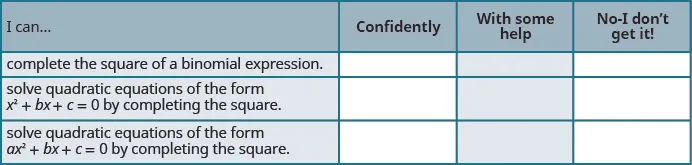After reviewing this checklist, what will you do to become confident for all objectives?

Order a print copy

As an Amazon Associate we earn from qualifying purchases.